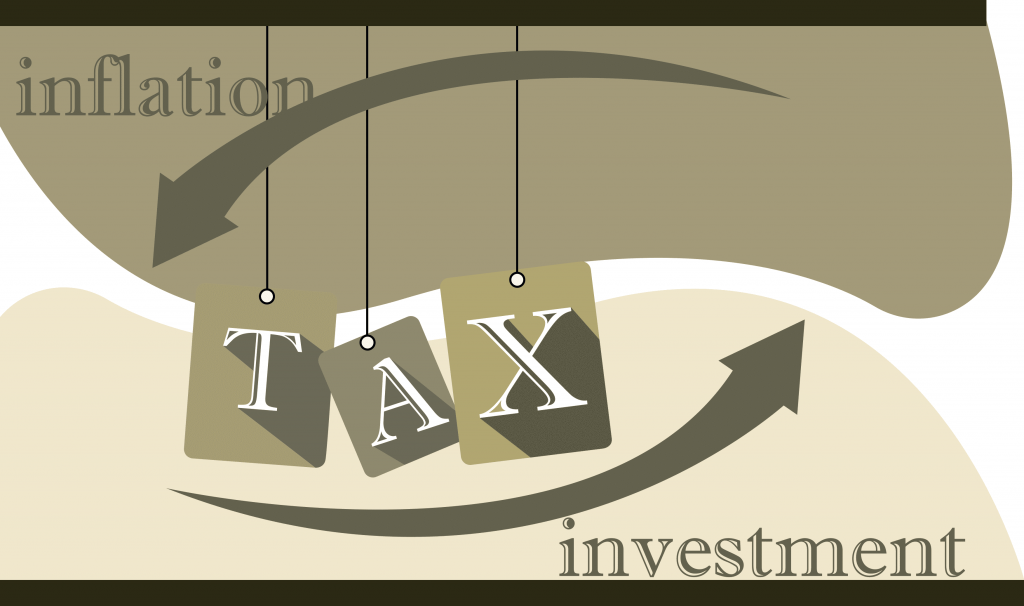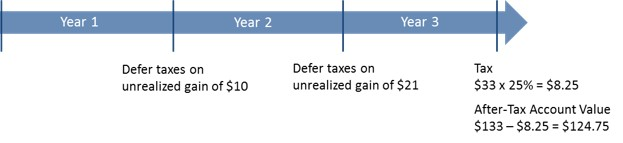# Effect of Taxes vs. Inflation on Asset Growth

## While the effect of taxes on investment return occurs when a sale transaction happens, the effect of inflation is continuous and is modeled as a discount factor.

Consider a scenario in which you invest \$100 in a financial instrument that promises a 10% annual return on a compounded basis. You sell your investment after holding it for 3 years. Let us now analyze how capital gains tax and inflation affects ROI (Return on Investment) for this transaction.The account value is growing at a 10% CAGR (Compound Annual Growth Rate). Compounded growth means the interest portion is also earning interest. For example, the \$10 interest earned at the end of the first year earns an interest of \$1 at the end of second year, contributing to the account growth in addition to the simple interest earned by the principal amount of the initial investment. Refer to “Power of Compounding” article to get a more detailed look on the effects of compounding.

Taxes are owed when an investment is finally sold and a profit is made. This profit is also called realized gain. Prior to the sale, any profit on paper is called unrealized gain. Though tax liability accrues on unrealized gain, actual tax payment is deferred until after the sale is made.The total tax is \$8.25 on a realized gain of \$33 assuming a 15% capital gains tax and a 10% state tax. Taxes reduce account value to \$124.75 and ROI to 7.6%1. In other words, After-Tax ROI is ROI calculated using After-Tax Account Value.

Let us see how inflation factors into the equation now. Inflation is the silent killer that lurks in the background, constantly eroding wealth by reducing its purchase power. While tax is only owed on the generated profit, inflation is adjusted on the entire net account value.

The Fed’s target rate for inflation is 2%. The effect of inflation can be modeled using a discount factor that is calculated based on the investment holding period. The discount factor is applied to the After-Tax Account Value. In our example, inflation further reduces the account value to \$117.41 and ROI to 5.5%1. Hence, Inflation-Adjusted ROI is After-Tax ROI less Inflation rate.

Both taxes and inflation reduce an investment’s ROI, and hence need to be considered when calculating the Real ROI.

Note:
1 ROI is calculated using the compound interest formula, Ending Balance (EB) = Beginning Balance (BB) x (1 + ROI)N

We specialize in tax-free retirement strategy and investments such as IUL, Annuity and LTC. Prefer a quick and complimentary consultation? Just email us at Karthik@FinCrafters.com